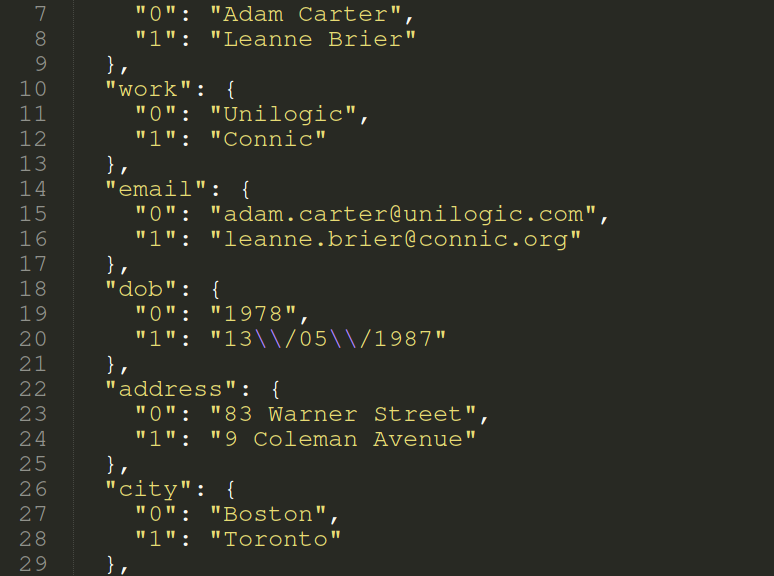# Pandas to SQL: Flatten JSON from Requests using Pandas (Multiple methods!)

Converting JSON to MySQL can be achieved in multiple ways, in this article we will look at three important ways to achieve this.

You can directly copy and paste these examples into your Python terminal. The reqres URL returns dummy JSON data.

```# generate fake json array for this example
import pandas as pd
import requests

r = requests.get('https://gist.githubusercontent.com/alejandro/1244310/raw/7a392a0c4a739602e80ba890e3a1dbee79183bd0/dummy.json')
jsonRaw = r.content
jsonDict = r.json()
print(jsonDict)
```

### Flatten JSON array using pd.json_normalize()

```import pandas as pd
import requests

jsonDict = {
"users": [
{
"id": 0,
"work": "Unilogic",
"email": "[email protected]",
"dob": "1978",
"city": "Boston",
"optedin": "true"
},
{
"id": 1,
"name": "Leanne Brier",
"work": "Connic",
"email": "[email protected]",
"dob": "13/05/1987",
"city": "Toronto",
"optedin": "false"
}
],
"images": [
"img0.png",
"img1.png",
"img2.png"
],
"coordinates": {
"x": 35.12,
"y": -21.49
},
"price": "\$59,395"
}
df = pd.json_normalize(jsonDict)
print(df)

df2 = pd.json_normalize(jsonDict['users'])
print(df2

```

In the above example you can see the problem with normalizing this array.

The actual dataframe is a list of dictionaries.

If we use dict[‘key’] then it works perfectly, but let’s try another method.

### Flatten using an awesome flattening module by amirziai. Install using pip install flatten_json

```import pandas as pd
import requests
from flatten_json import flatten

jsonDict = ...

flatDict = flatten(jsonDict)

json.dumps(flatDict)```

As you can probably already tell, this didn’t work correctly for our schema.

That’s because our data is technically 3 unrelated json arrays.

“price” has no relation to “users”

Let’s make a small change.

```jsonDict = ...

flatKeyed = (flatten(d) for d in jsonDict['users'])
df = pd.DataFrame(flatKeyed)
df.to_json()
```

# Perfect!

### Flatten JSON and import into SQL Table

```from sqlalchemy import create_engine
import pymysql
import pandas as pd
import requests
from flatten_json import flatten

class SqlWorker(object):
""" df, host, user, pass, dbase, table"""
def __init__(self, arg, dbHost, dbUser, dbPass, dbDbse, dbTble):
self.arg = arg
print(self.arg)
self.dbUser = dbUser
self.dbPass = dbPass
self.dbHost = dbHost
self.dbDbse = dbDbse
self.dbTble = dbTble
self.engine = create_engine(
'mysql+pymysql://'+self.dbUser+':'+self.dbPass+'@'+self.dbHost+'/'+self.dbDbse, pool_recycle=3600)
self.connection = self.engine.connect()
# self.frame = self.arg.to_sql(name=self.dbTble, con=self.connection, if_exists='append')
try:
self.frame = self.arg.to_sql(name=self.dbTble, con=self.connection, if_exists='append')
except ValueError as vx:
print(vx)
except Exception as ex:
print(ex)
else:
print("Table %s created successfully."%self.dbTble)
finally:
self.connection.close()

jsonDict = ...

flatKeyed = (flatten(d) for d in jsonDict['users'])
df = pd.DataFrame(flatKeyed)
SqlWorker(df, 'localhost', 'root', 'root', 'users_db', 'usertable')
```